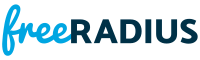channel.c File ReferenceInclude dependency graph for channel.c:

Go to the source code of this file.

Data Structures

struct  rchannel_t

Typedefs

typedef struct rchannel_t rchannel_t

Functions

ssize_t fr_channel_drain (int fd, fr_channel_type_t *pchannel, void *inbuf, size_t buflen, uint8_t **outbuf, size_t have_read)

ssize_t fr_channel_read (int fd, fr_channel_type_t *pchannel, void *inbuf, size_t buflen)

ssize_t fr_channel_write (int fd, fr_channel_type_t channel, void const *inbuf, size_t buflen)

static ssize_t lo_read (int fd, void *inbuf, size_t buflen)

static ssize_t lo_write (int fd, void const *inbuf, size_t buflen)

Data Structure Documentation

 struct rchannel_t

Definition at line 29 of file channel.c.

Data Fields
uint32_t channel
uint32_t length

Typedef Documentation

 typedef struct rchannel_t rchannel_t

Function Documentation

 ssize_t fr_channel_drain ( int fd, fr_channel_type_t * pchannel, void * inbuf, size_t buflen, uint8_t ** outbuf, size_t have_read )

Definition at line 61 of file channel.c.Here is the call graph for this function:Here is the caller graph for this function:

 ssize_t fr_channel_read ( int fd, fr_channel_type_t * pchannel, void * inbuf, size_t buflen )

Definition at line 125 of file channel.c.Here is the call graph for this function:Here is the caller graph for this function:

 ssize_t fr_channel_write ( int fd, fr_channel_type_t channel, void const * inbuf, size_t buflen )

Definition at line 205 of file channel.c.Here is the call graph for this function:Here is the caller graph for this function:

 static ssize_t lo_read ( int fd, void * inbuf, size_t buflen )
static

Definition at line 35 of file channel.c.Here is the caller graph for this function:

 static ssize_t lo_write ( int fd, void const * inbuf, size_t buflen )
static

Definition at line 177 of file channel.c.Here is the caller graph for this function: